## ML Aggarwal Class 10 Solutions for ICSE Maths Chapter 25 Value Added Tax Chapter Test

These Solutions are part of ML Aggarwal Class 10 Solutions for ICSE Maths. Here we have given ML Aggarwal Class 10 Solutions for ICSE Maths Chapter 1 Value Added Tax Chapter Test.

Question 1.
A shopkeeper bought a washing machine at a discount of 20% from a wholesaler, the printed price of the washing machine being ₹ 18000. The shopkeeper sells it to a consumer at a discount of 10% on the printed price. If the rate of sales tax is 8%, find:
(i) the VAT paid by the shopkeeper.
(ii) the total amount that the consumer pays for the washing machine.
Solution:
(i) SP of washing machineQuestion 2.
A manufacturing company sold an article to its distributor for ₹22000 including VAT. The distributor sold the article to a dealer for ₹22000 excluding tax and the dealer sold it to a consumer for ₹25000 plus tax (under VAT). If the rate of sales tax (under VAT) at each stage is 10%, find :
(i) the sale price of the article for the manufacturing company.
(ii) the amount of VAT paid by the dealer.
Solution:Question 3.
The marked price of an article is ₹7500. A shopkeeper sells the article to a consumer at the marked prices and charges sales tax at . the rate of 7%. If the shopkeeper pays a VAT of ₹105, find the price inclusive of sales tax of the article which the shopkeeper paid to the wholesaler.
Solution:Question 4.
A shopkeeper buys an article at a discount of 30% and pays sales tax at the rate of 6%. The shopkeeper sells the article to a consumer at 10% discount on the list price and charges sales tax at the’ same rate. If the list price of the article is ₹3000, find the price inclusive of sales tax paid by the shopkeeper.
Solution:Question 5.
Mukerjee purchased a movie camera for ₹27468. which includes 10% rebate on the list price and then 9% sales tax (under VAT) on the remaining price. Find the list price of the movie camera.
Solution:Question 6.
A retailer buys an article at a discount of 15% on the printed price from a wholesaler. He marks up the price by 10%. Due to competition in the market, he allows a discount of 5% to a buyer. If the buyer pays ₹451.44 for the article inclusive of sales tax (under VAT) at 8%, find :
(i) the printed price of the article
(ii) the profit percentage of the retailer.
Solution:Hope given ML Aggarwal Class 10 Solutions for ICSE Maths Chapter 1 Value Added Tax Chapter Test are helpful to complete your math homework.

If you have any doubts, please comment below. APlusTopper try to provide online math tutoring for you.

## ML Aggarwal Class 10 Solutions for ICSE Maths Chapter 24 Probability Chapter Test

These Solutions are part of ML Aggarwal Class 10 Solutions for ICSE Maths. Here we have given ML Aggarwal Class 10 Solutions for ICSE Maths Chapter 24 Probability Chapter Test.

Question 1.
A game consists of spinning an arrow which comes to rest at one of the regions 1, 2 or 3 (shown in the given figure). Are the outcomes 1, 2 and 3 equally likely to occur? Give reasons.Solution: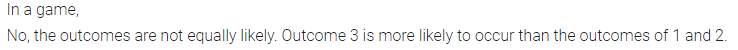Question 2.
In a single throw of a die, find the probability of getting
(i) a number greater than 5
(ii) an odd prime number
(iii) a number which is multiple of 3 or 4.
Solution:Question 3.
A lot consists of 144 ball pens of which 20 are defective and the others are good. Rohana will buy a pen if it is good, but will not buy it if it is defective. The shopkeeper draws one pen at random and gives it to her. What is the probability that :
(i) She will buy it ?
(ii) She will not buy it ?
Solution:
In a lot, there are 144 ball pens in which defective ball pens are = 20
and good ball pens are = 144 – 20 = 124
Rohana buys a pen which is good only.
(i) Now the number of possible outcomes = 144
and the number of favourable outcomes = 124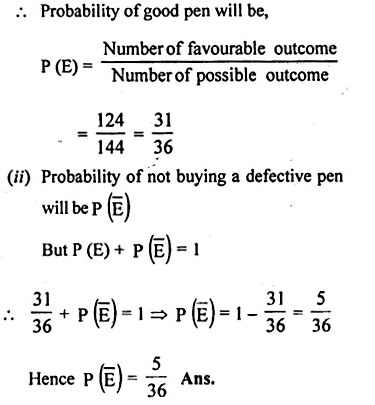Question 4.
A lot consists of 48 mobile phones of which 42 are good, 3 have only minor defects and 3 have major defects. Varnika will buy a phone if it is good but the trader will only buy a mobile if it has no major defect. One phone is selected at random from the lot. What is the probability that it is
(i) acceptable to Varnika?
(ii) acceptable to the trader?
Solution: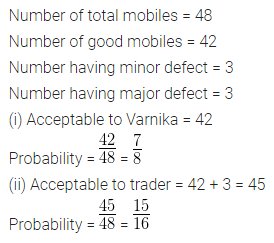Question 5.
A bag contains 6 red, 5 black and 4 white balls. A ball is drawn from the bag at random. Find the probability that the ball drawn is
(i) white
(ii) red
(iii) not black
(iv) red or white.
Solution: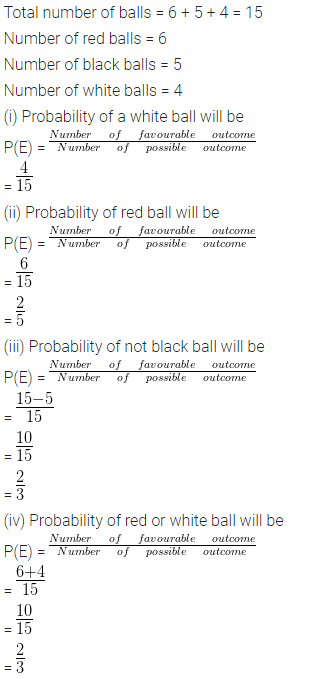Question 6.
A bag contains 5 red, 8 white and 7 black balls. A ball is drawn from the bag at random. Find the probability that the drawn ball is:
(i) red or white
(ii) not black
(iii) neither white nor black
Solution: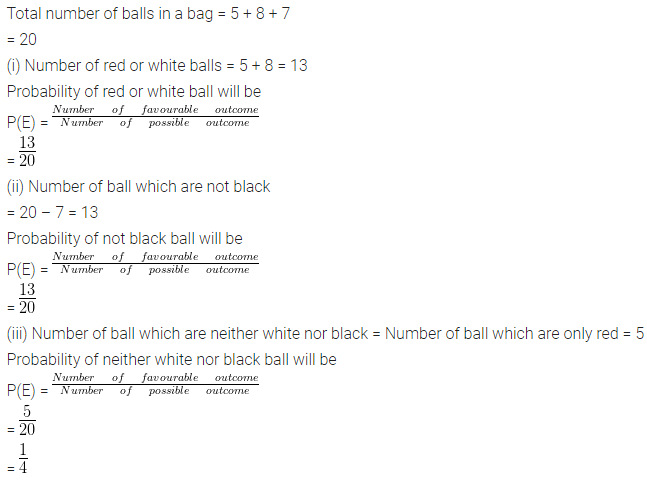Question 7.
A bag contains 5 white balls, 7 red balls, 4 black balls and 2 blue balls. One ball is drawn at random from the bag. What is the probability that the ball drawn is :
(i) white or blue
(ii) red or black
(iii) not white
(iv) neither white nor black ?
Solution:Question 8.
A box contains 20 balls bearing numbers 1, 2, 3, 4,……, 20. A ball is drawn at random from the box. What is the probability that the number on the ball is
(i) an odd number
(ii) divisible by 2 or 3
(iii) prime number
(iv) not divisible by 10 ?
Solution:Question 9.
Find the probability that a number selected at random from the numbers 1, 2, 3,……35 is a
(i) prime number
(ii) multiple of 7
(iii) multiple of 3 or 5.
Solution:Question 10.
Cards marked with numbers 13, 14, 15,…..60 are placed in a box and mixed thoroughly. One card is drawn at random from the box. Find the probability that the number on the card is
(i) divisible by 5
(ii) a number which is a perfect square.
Solution: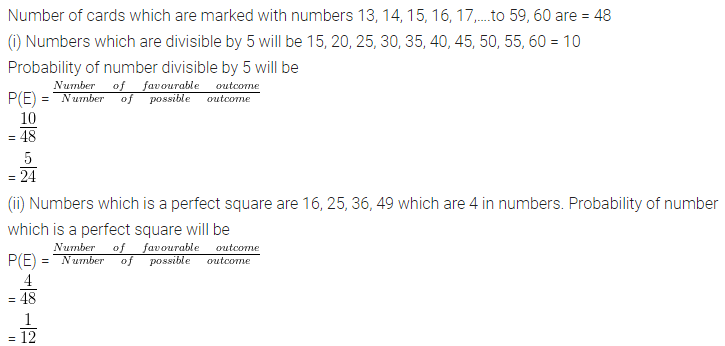Question 11.
The box has cards numbered 14 to 99. Cards are mixed thoroughly and a card is drawn at random from the box. Find the probability that the card drawn from the box has
(i) an odd number
(ii) a perfect square number.
Solution:Question 12.
A bag contains 5 red balls and some blue balls. If the probability of drawing a blue ball is four times that of a red ball, find the number of balls in the bags.
Solution: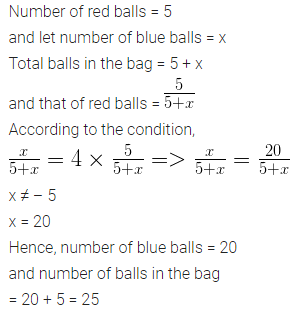Question 13.
A bag contains 18 balls out of which x balls are white.
(i) If one ball is drawn at random from the bag, what is the probability that it is white ball?
(ii) If 2 more white balls are put in the bag, the probability of drawing a white ball will be $$\\ \frac { 9 }{ 8 }$$ times that of probability of white ball coming in part (i). Find the value of x.
Solution:
Total numbers of balls in a bag = 18
No. of white balls = x
(i) One ball is drawn a random
Probability of being a white ball = $$\\ \frac { x }{ 18 }$$
(ii) If 2 more white balls an put, then number of white balls = x + 2
and probability is $$\\ \frac { 9 }{ 8 }$$ timesQuestion 14.
A card is drawn from a well-shuffled pack of 52 cards. Find the probability that the card drawn is :
(i) a red face card
(ii) neither a club nor a spade
(iii) neither an ace nor a king of red colour
(iv) neither a red card nor a queen
(v) neither a red card nor a black king.
Solution:Question 15.
From pack of 52 playing cards, blackjacks, black kings and black aces are removed and then the remaining pack is well-shuffled. A card is drawn at random from the remaining pack. Find the probability of getting
(i) a red card
(ii) a face card
(iii) a diamond or a club
(iv) a queen or a spade.
Solution: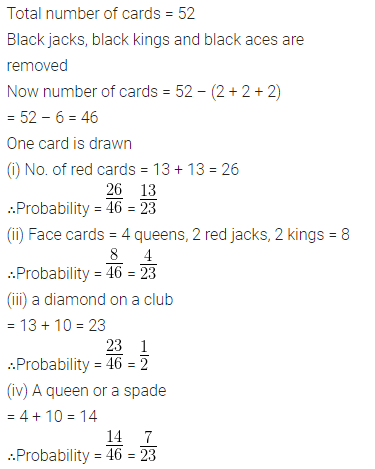Question 16.
Two different dice are thrown simultaneously. Find the probability of getting:
(i) sum 7
(ii) sum ≤ 3
(iii) sum ≤ 10
Solution: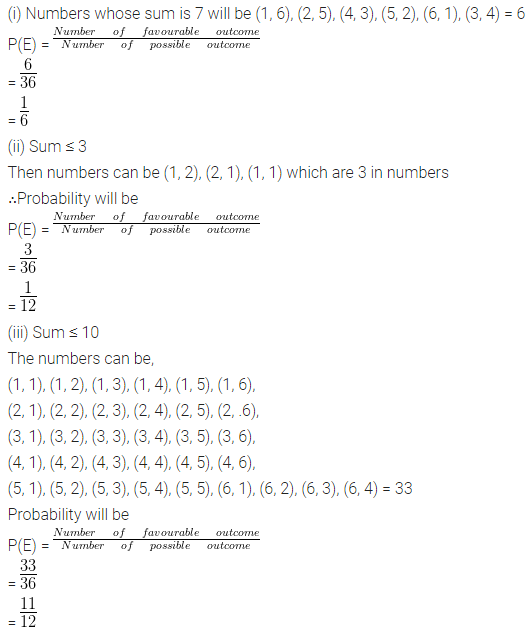Question 17.
Two dice are thrown together. Find the probability that the product of the numbers on the top of two dice is
(i) 6
(ii) 12
(iii) 7
Solution: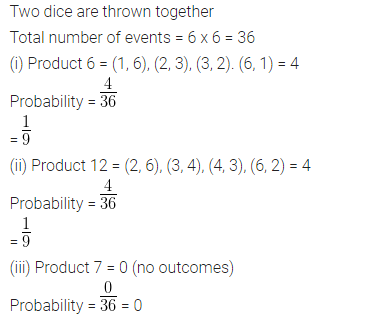We hope the ML Aggarwal Class 10 Solutions for ICSE Maths Chapter 24 Probability Chapter Test help you. If you have any query regarding ML Aggarwal Class 10 Solutions for ICSE Maths Chapter 24 Probability Chapter Test, drop a comment below and we will get back to you at the earliest.

## ML Aggarwal Class 10 Solutions for ICSE Maths Chapter 23 Measures of Central Tendency Chapter Test

These Solutions are part of ML Aggarwal Class 10 Solutions for ICSE Maths. Here we have given ML Aggarwal Class 10 Solutions for ICSE Maths Chapter 23 Measures of Central Tendency Chapter Test.

Question 1.
Arun scored 36 marks in English, 44 marks in Civics, 75 marks in Mathematics and x marks in Science. If he has scored an average of 50 marks, find x.
Solution:Question 2.
The mean of 20 numbers is 18. If 3 is added to each of the first ten numbers, find the mean of new set of 20 numbers.
Solution: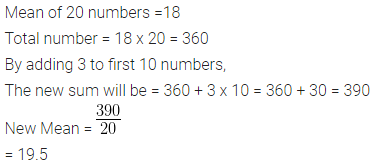Question 3.
The average height of 30 students is 150 cm. It was detected later that one value of 165 cm was wrongly copied as 135 cm for computation of mean. Find the correct mean.
Solution:Question 4.
There are 50 students in a class of which 40 are boys and the rest girls. The average weight of the students in the class is 44 kg and average weight of the girls is 40 kg. Find the average weight of boys.
Solution:Question 5.
The contents of 50 boxes of matches were counted giving the following resultsCalculate the mean number of matches per box.
Solution: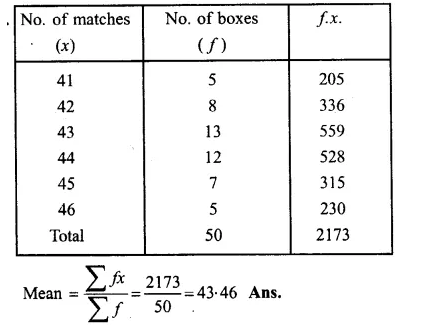Question 6.
The heights of 50 children were measured (correct to the nearest cm) giving the following results :Solution:Question 7.
Find the value of p for the following distribution whose mean is 20.6 :Solution:Question 8.
Find the value of p if the mean of the following distribution is 18.Solution:Question 9.
Find the mean age in years from the frequency distribution given below:Solution:Question 10.
Calculate the Arithmetic mean, correct to one decimal place, for the following frequency distribution :Solution: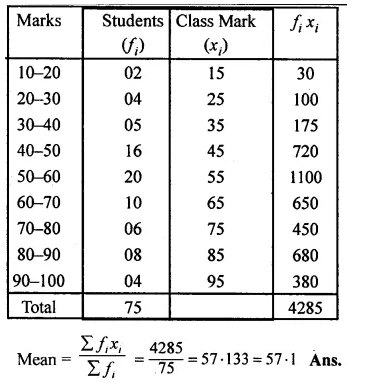Question 11.
The mean of the following frequency distribution is 62.8. Find the value of p.Solution:Hence p = 10

Question 12.
The daily expenditure of 100 families are given below. Calculate f1, and f2, if the mean daily expenditure is Rs 188.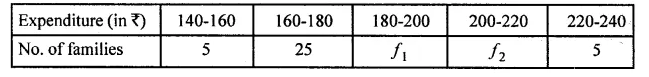Solution:Question 13.
The measures of the diameter of the heads of 150 screw is given in the following table. If the mean diameter of the heads of the screws is 51.2 mm, find the values of p and q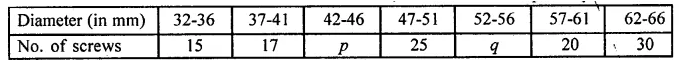Solution:
Mean = 51.2
No. of screws = 150Question 14.
The median of the following numbers, arranged in ascending order is 25. Find x, 11, 13, 15, 19, x + 2, x + 4, 30, 35, 39, 46
Solution:
Here, n = 10, which is evenQuestion 15.
If the median of 5, 9, 11, 3, 4, x, 8 is 6, find the value of x.
Solution: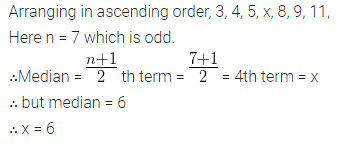Question 16.
Find the median of: 17, 26, 60, 45, 33, 32, 29, 34, 56 If 26 is replaced by 62, find the new median.
Solution:Question 17.
The marks scored by 16 students in a class test are : 3, 6, 8, 13, 15, 5, 21, 23, 17, 10, 9, 1, 20, 21, 18, 12
Find
(i) the median
(ii) lower quartile
(iii) upper quartile
Solution:
Arranging the given data in ascending order:
1, 3, 5, 6, 8, 9, 10, 12, 13, 15, 17, 18, 20, 21, 21, 23
Here n = 16 which is even.Question 18.
Find the median and mode for the set of numbers : 2, 2, 3, 5, 5, 5, 6, 8, 9
Solution:Question 19.
Calculate the mean, the median and the mode of the following distribution :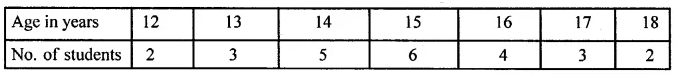Solution:Question 20.
The daily wages of 30 employees in an establishment are distributed as follows :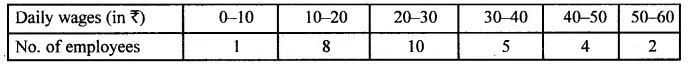Estimate the modal daily wages for this distribution by a graphical method.
Solution: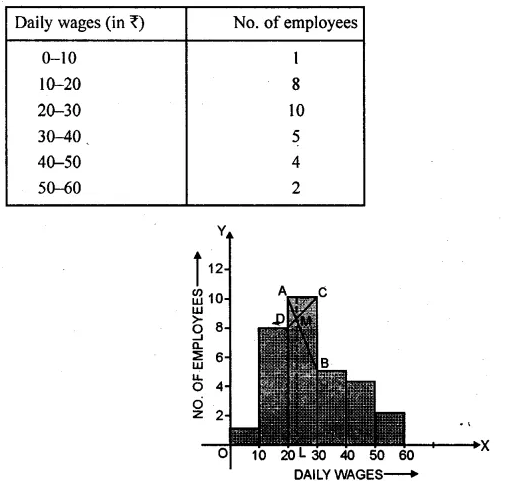Question 21.
Using the data given below, construct the cumulative frequency table and draw the ogive. From the ogive, estimate ;
(i) the median
(ii) the inter quartile range.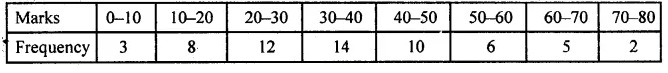Also state the median class
Solution: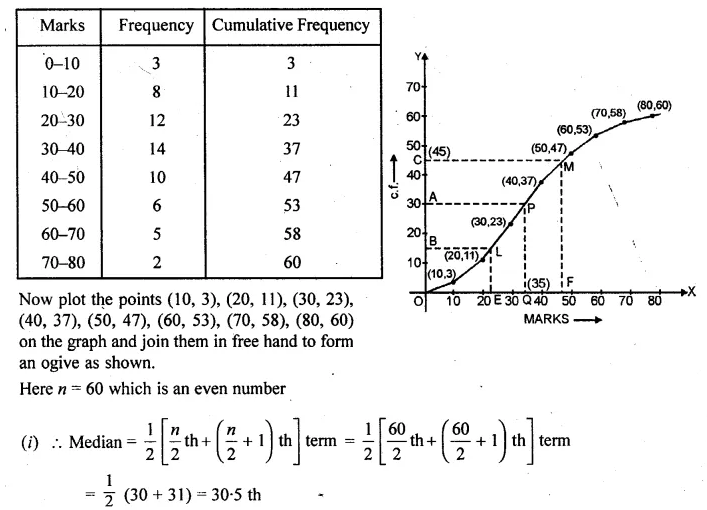Question 22.
Draw a cumulative frequency curve for the following data :Hence determine:
(i) the median
(ii) the pass marks if 85% of the students pass.
(iii) the marks which 45% of the students exceed.
Solution: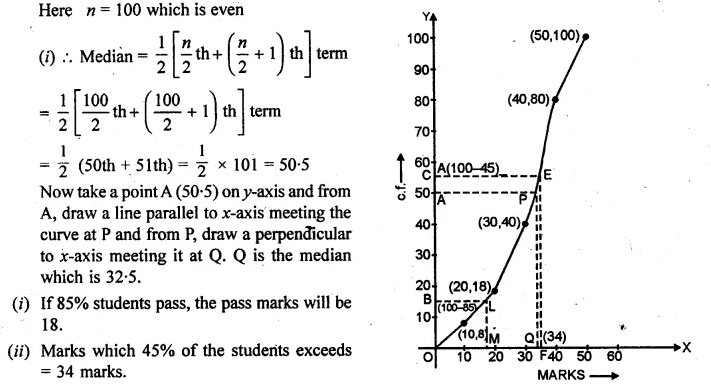We hope the ML Aggarwal Class 10 Solutions for ICSE Maths Chapter 23 Measures of Central Tendency Chapter Test help you. If you have any query regarding ML Aggarwal Class 10 Solutions for ICSE Maths Chapter 23 Measures of Central Tendency Chapter Test, drop a comment below and we will get back to you at the earliest.

## ML Aggarwal Class 10 Solutions for ICSE Maths Chapter 21 Heights and Distances Chapter Test

These Solutions are part of ML Aggarwal Class 10 Solutions for ICSE Maths. Here we have given ML Aggarwal Class 10 Solutions for ICSE Maths Chapter 21 Heights and Distances Chapter Test.

Question 1.
The angle of elevation of the top of a tower from a point A (on the ground) is 30°. On walking 50 m towards the tower, the angle of elevation is found to be 60°. Calculate
(i) the height of the tower (correct to one decimal place).
(ii) the distance of the tower from A.
Solution: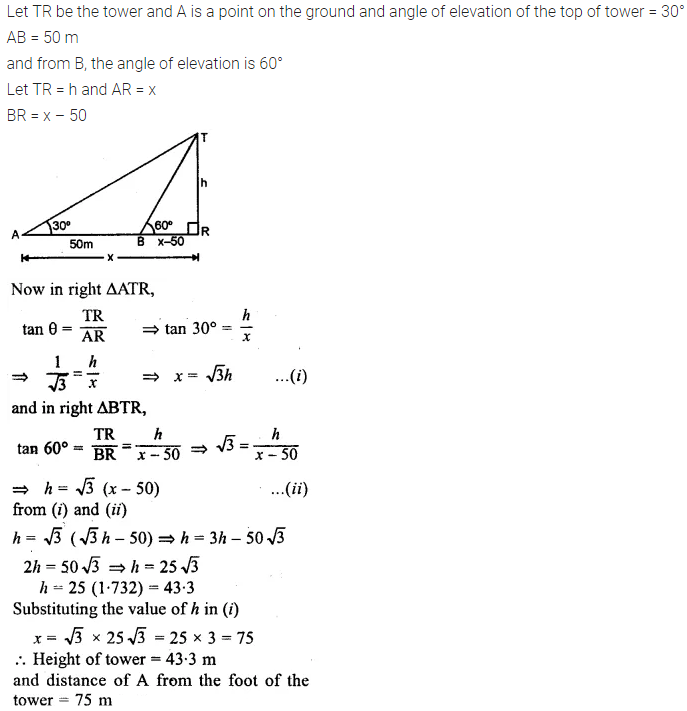Question 2.
An aeroplane 3000 m high passes vertically above another aeroplane at an instant when the angles of elevation of the two aeroplanes from the same point on the ground are 60° and 45° respectively. Find the vertical distance between the two planes.
Solution:Question 3.
A 7m long flagstaff is fixed on the top of a tower. From a point on the ground, the angles of elevation of the top and bottom of the flagstaff are 45° and 36° respectively. Find the height of the tower correct to one place of demical.
Solution: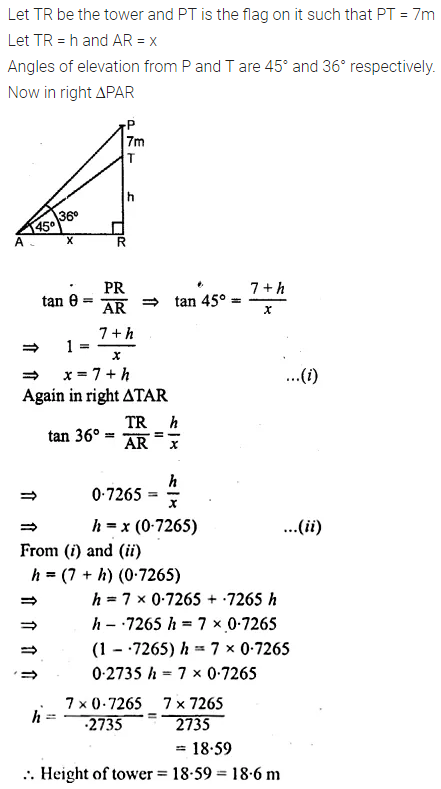Question 4.
A boy 1.6 m tall is 20 m away from a tower and observes that the angle of elevation of the top of the tower is 60°. Find the height of the tower.
Solution:Question 5.
A boy 1.54 m tall can just see the sun over a wall 3.64 m high which is 2.1 m away from him. Find the angle of elevation of the sun.
Solution: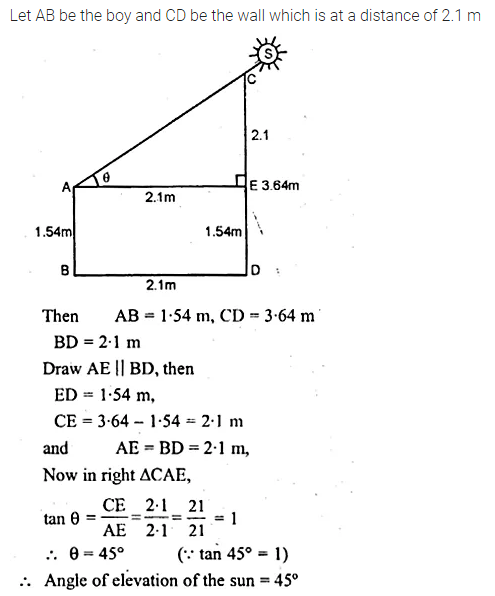Question 6.
In the adjoining figure, the angle of elevation of the top P of a vertical tower from a point X is 60° ; at a point Y, 40 m vertically above X, the angle of elevation is 45°. Find
(i) the height of the tower PQ
(ii) the distance XQ
(Give your answer to the nearest metre)Solution:Question 7.
An aeroplane is flying horizontally 1 km above the ground is observed at an elevation of 60°. After 10 seconds, its elevation is observed to be 30°. Find the speed of the aeroplane in km/hr.
Solution: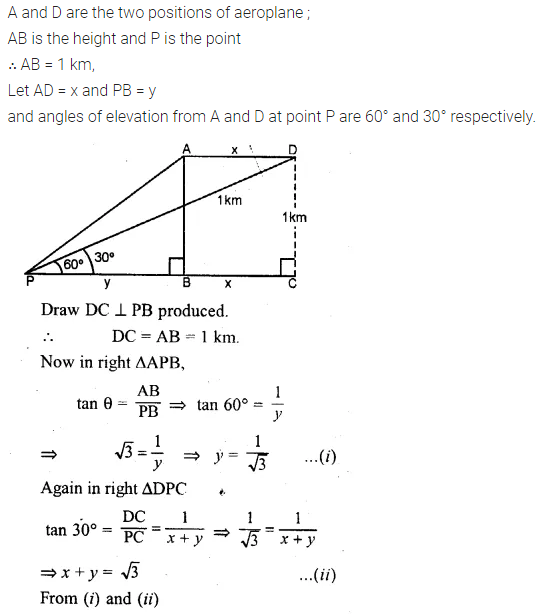Question 8.
A man on the deck of a ship is 16 m above the water level. He observes that the angle of elevation of the top of a cliff is 45° and the angle of depression of the base is 30°. Calculate the distance of the cliff from the ship and the height of the cliff.
Solution:Question 9.
There is a small island in between a river 100 metres wide. A tall tree stands on the island. P and Q are points directly opposite to each other on the two banks and in the line with the tree. If the angles of elevation of the top of the tree from P and Q are 30° and 45° respectively, find the height of the tree.
Solution: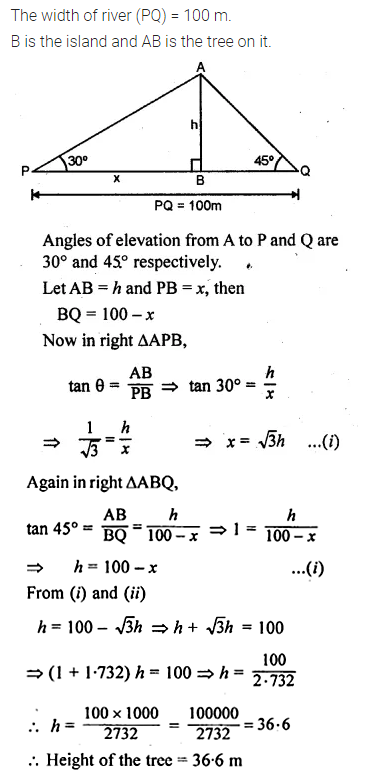Question 10.
A man standing on the deck of the ship which is 20 m above the sea-level, observes the angle of elevation of a bird as 30° and the angle of depression of its reflection in the sea as 60°. Find the height of the bird
Solution:We hope the ML Aggarwal Class 10 Solutions for ICSE Maths Chapter 21 Heights and Distances Chapter Test help you. If you have any query regarding ML Aggarwal Class 10 Solutions for ICSE Maths Chapter 21 Heights and Distances Chapter Test, drop a comment below and we will get back to you at the earliest.

## ML Aggarwal Class 10 Solutions for ICSE Maths Chapter 20 Trigonometric Tables Chapter Test

These Solutions are part of ML Aggarwal Class 10 Solutions for ICSE Maths. Here we have given ML Aggarwal Class 10 Solutions for ICSE Maths Chapter 19 Trigonometric Tables Chapter Test.

Question 1.
Using trigonometrical tables, find the values of :
(i) sin 48° 52′
(ii) cos 37° 34′
(iii) tan 18° 21′.
Solution:Question 2.
Use tables to find the acute angle θ, given that
(i) sin θ = 0.5766
(ii) cos θ = 0.2495
(iii) tan θ = 2.4523.
Solution:Question 3.
If θ is acute and cos θ = 0.53, find the value of tan θ.
Solution:Question 4.
Find the value of: sin 22° 11′ + cos 57° 20′ – 2 tan 9° 9′.
Solution:Question 5.
If θ is acute and sin θ = 0.7547, find the value of: (i) θ (ii) cos θ (iii) 2 cos θ – 3 tan θ.
Solution:We hope the ML Aggarwal Class 10 Solutions for ICSE Maths Chapter 20 Trigonometric Tables Chapter Test help you. If you have any query regarding ML Aggarwal Class 10 Solutions for ICSE Maths Chapter 20 Trigonometric Tables Chapter Test, drop a comment below and we will get back to you at the earliest.